Press "Enter" to skip to content

#• Motion is change in position of an object with time. When this change in position is along a straight line then we consider it motion in a straight line.
• Everything in this universe is characterized by motion in anyway round.
• Kinematics, a branch of physics which deals with the study of motion.
• Many phenomena associated with nature shows motion in one way or the other, as leaves falling from trees, movement of water etc.
• In this article, we have provided you with the notes of motion in a straight line of class 11.
• Motion in a straight line is also called rectilinear motion.

## Rest and Motion:

• When the position of an object is changing with respect to a frame of reference (say S) then the object is said to be in motion with respect to that frame of reference.
• When the position of an object is stable (not changing) with respect to a frame of reference (say S) then the object is said to be in rest with respect to that frame of reference.
• Absolute rest and absolute motion is not possible in this universe.
• Rest and motion are relative terms both goes hand in hand.

## Types of motion:

There are mainly three types of motion as commonly observed:

1. Rectilinear and Translatory motion
2. Circular and Rotatory motion
3. Oscillatory and Vibratory motion

The actual concept of motion in a straight line is in Rectilinear motion or 1-D motion. Learn it wisely.

## 1.   Rectilinear motion:

• Motion of an object in a straight line is said to be rectilinear motion.
• It is also often referred to as straight motion or rectilinear kinematics.

## Parameters of rectilinear motion:

There are several parameters involved in motion in a straight line (rectilinear motion). They are time, position, distance, velocity etc. Here is the detailed analysis please read in detail below:

Time:

• Time is an essential parameter while studying motion.
• It is scalar quantity.
• Its SI unit is Second.
• The universal law about time is that it never decreases.
• At a certain instant of time any object can be at one place only.Position:

• It is the place defined with respect to any reference point (in a frame of reference).
• It is a vector quantity but distance is scalar.
• Generally, we define position with respect to origin (O). So we write position of A and B with respect to origin is as r1 = $$\vec{OA}$$ and r2 = $$\vec{OB}$$ respectively.

Distance:

• The length of actual path travelled by any object is said to be distance.
• In the figure above, the length of the total path ACB is the distance.
• The SI unit of distance is metre.
• It is a scalar quantity.
• Distance also never decreases with time.

Displacement:

• When an object travels from one point to other then the shortest length of path between two points is said to be displacement.
• Or simply, the change in position vector of an object in the given time interval is said to be displacement.
$$\vec{AB}$$ =  $$\vec{r}$$ = $$\vec{r_2}$$ – $$\vec{r_1}$$
• It is a vector quantity.
• Its SI unit is also metre.
• Displacement can decrease with time.
• Motion in a straight line has displacement equal to that of distance.

### Anomaly of distance and displacement:

• Displacement can be positive, negative or zero, but distance will always be positive.
• Distance is always greater than or equal to the displacement. I.e. Distance ≥ Displacement.
• When a particle is moving along a straight line then distance will be equal to the displacement.
• Distance is scalar as it has only magnitude but displacement is a vector quantity as it has both magnitude as well as direction.

Average speed:

• Total distance covered by an object when divided by total time taken to cover that distance is called average speed.
• Average speed = $$\frac{total\:distance\:travelled}{Total\:time\:taken}$$ = $$\frac{∆s}{∆t}$$
• It is a scalar quantity.
• Its SI unit is m/s.

Uniform Speed:

• If an object covers equal distances in equal intervals of time than the speed of the moving object is called uniform speed.
• In this type of motion, a position-time graph is always a straight line.

Average Velocity:

• The displacement, when divided by total time taken for that displacement, gives us average velocity.
• Average velocity = $$\frac{Displacement}{Total\:time\:taken}$$ = $$\frac{∆\vec{r}}{∆t}$$
• It is a vector quantity.
• Its dimensional formula is [M0L1-1].
• Its SI unit is also m/s.

### Anomaly of Average speed and Average velocity:

• Average velocity is always single-valued function whereas Average speed can be many-valued functions.
• Average speed will always be positive whereas average velocity can be positive, negative or zero.

Instantaneous speed:

• Instantaneous speed is the speed at any particular instant of time.
• The ratio of total distance travelled to the time taken is instantaneous speed. But the condition is that the time interval should be infinitesimally (extremely) small.
• It may be totally different from average speed.
• Mathematically, we express instantaneous speed as v = $$\lim_{∆t\to0}$$ $$\frac{∆s}{∆t}$$ = $$\frac{ds}{dt}$$

Instantaneous velocity:

• The Velocity of any object at any particular instant of time is said to be instantaneous velocity.
• The ratio of total displacement to the time taken is instantaneous velocity. But the condition is that the time interval should be infinitesimally (extremely) small.
• It may be totally different from average velocity.
• Mathematically, we express it as v = $$\lim_{∆t\to0}$$ $$\frac{∆r}{∆t}$$ = $$\frac{dr}{dt}$$

Remember: The magnitude of average velocity |Vavg| and average speed may not be equal but instantaneous velocity |V| is always equal to instantaneous speed.

## Acceleration:

• The rate of change of velocity with time is said to be acceleration.
• Acceleration = $$\frac{change\:in\:velocity}{time\:taken}$$
e. a = $$\frac{∆v}{∆t}$$ = $$\frac{v-u}{t}$$
Where v and u are final and initial velocity respectively.
• Its unit is m/s2.
• Its dimensional formula is [M0LT-2]
• Acceleration is a vector quantity.
• Acceleration can be positive negative or zero.
Positive acceleration – Velocity increasing with time.
Zero acceleration – Velocity is uniform with time.
Negative acceleration (Retardation) – velocity decrease with time.

In this section, we will only learn about uniformly accelerated motion in a straight line problems and concepts.

### A.   Average acceleration:

• If a particle is accelerated with acceleration a1 for a time t1 and a2 for tome t2 then average acceleration is defined as:

aavg = $$\frac{a_{1}t_{1}\:+\: a_{2}t_{2}}{t_{1}\: +\:t_{2}}$$

### B.   Uniform acceleration:

• When any object undergoes equal changes in velocity in equal time intervals it is called uniform acceleration.

### C.   Instantaneous acceleration:

• The acceleration of an object at a particular specific moment of time is said to be instantaneous acceleration.
• Mathematically, the limit of average acceleration as the time interval approaches zero is known to be instantaneous acceleration.
$$\vec{a}$$ = $$\lim_{∆t\to0}$$ $$\frac{∆{\vec{v}}}{∆t}$$ = $$\frac{dv}{dt}$$

## Equations for uniformly accelerated motion in a straight line:

If a body starts with some velocity and after some time its velocity changes and the body being uniformly accelerated, then there are some relations obtained between its velocity, distance travelled, time taken etc. (only for motion in a straight line).

Let, Initial velocity = u
Final velocity = v
Time taken = t
Uniform acceleration = a
Distance travelled = s then,

1. v= u + at
2. s = ut + $$\frac{1}{2}$$ at2
3. v2 = u2 + 2as
4. Distance travelled in nth second is given by
S­n = u + $$\frac{a}{2(2n-1)}$$
5. If the body is moving with uniform acceleration then velocity of the body at mid-point of the path is $$\sqrt{u^{2} + v^{2}}$$

## Graphical representation of motion in a straight line :

1. ### Displacement-time graph:

“Slope of Displacement-Time graph gives us average velocity”.

• For stationary body:• For body moving with constant velocity: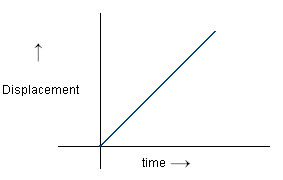• Body moving with constant acceleration:• Body moving with constant retardation:• When body moving with infinite velocity. Actually it is not possible.### 2. Velocity-Time Graph:

Slope of Velocity-Time graph gives average acceleration.”

• Velocity-Time graph for body moving with constant velocity.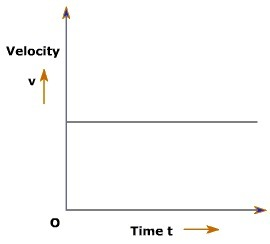• The Velocity-Time graph for body moving with constant acceleration:• Velocity-Time graph of body moving with constant retardation and its initial velocity is not zero.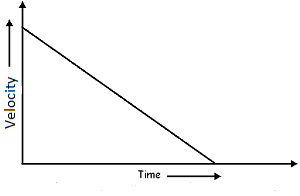• The Velocity-Time graph of body moving with increasing acceleration:• Velocity-Time graph of body moving with decreasing acceleration: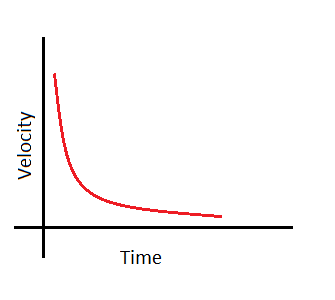### 3. Acceleration-Time graph:

• When body is moving with constant acceleration:• Acceleration-Time graph when body is moving with constant increasing acceleration: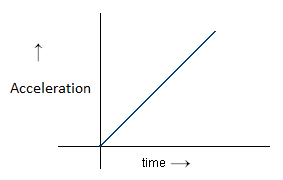• When body is moving with constant decreasing acceleration: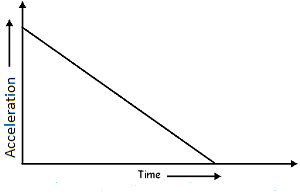## Motion under gravity:

• The equations of motion under gravity (free fall motion) are:
1. v = u + gt
2. h = ut + gt2
3. v2 = u2 + 2gh
• If the object is thrown upwards simply replace g by (-g) in the above equations. They are
1. v = u – gt
2. h = ut – gt2
3. v2 = u2 – 2gh

Some important formula for motion under gravity are:

• Time taken to reach maximum height
tA = $$\frac{u}{g}$$ = $$\sqrt{\frac{2h}{g}}$$
• Maximum height reached by the object:
hmax = $$\frac{u^2}{2g}$$

Note: The value of g is 9.8m/s2

## Relative velocity:

• The rate of change of position of one object with respect to the other is called relative velocity.
• Relative velocity of A with respect to B is written as VAB = VB ± V
• If both body A and B is moving in the same direction then VAB = VB – VA
• If Body A and B are moving in opposite direction then VAB = VA + VB
• When two bodies are moving at some angle then
VAB = $$\sqrt{{v}_{A}^{2}\: +\: {v}_{B}^{2} – 2V_{A}V_{B}cosɵ}$$

Practice Important Questions on Motion in a straight lineClick Here

Share with your Friends

## Be First to Comment

error: Content is protected !!